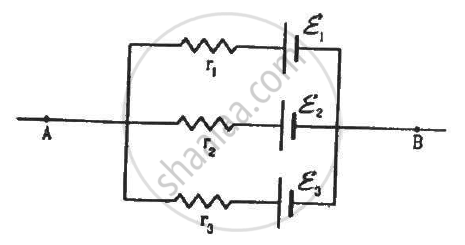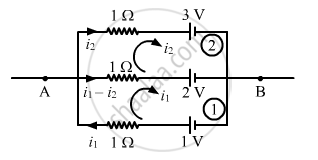Advertisement Remove all ads

# In the Circuit Shown in the Figure, ε1 = 3 V, ε2 = 2 V, εA = 1 V And R1 = R2 = R3 = 1ω. Find the Potential Difference Between the Points A And B And the Current Through Each Branch. - Physics

Sum

In the circuit shown in the figure, ε1 = 3 V, ε2 = 2 V, εa = 1 V and r1 = r2 = r3 = 1Ω. Find the potential difference between the points A and B and the current through each branch.Advertisement Remove all ads

#### SolutionApplying KVL in loop 1, we get:-

$i_1 + \left( i_1 - i_2 \right) - 1 + 2 = 0$

$2 i_1 - i_2 = - 1 .............(1)$

Similarly, for loop 2:-

$i_2 - \left( i_1 - i_2 \right) - 2 + 3 = 0$

$2 i_2 - i_1 = - 1 ............(2)$

Solving (1) and (2), we get:-

$i_1 = i_2 = 1 A$

Potential difference between A and B:-

$V_A - V_B = E_2 - \left( i_1 - i_2 \right) \times 1 = 2 - \left( 1 - 1 \right) = 2 V$

Current through the top branch = i2 = 1 A

Current through the middle branch = i1 - i2 = 0 A

Current through the bottom branch = i1 = 1 A

Concept: Potential Difference and Emf of a Cell
Is there an error in this question or solution?
Advertisement Remove all ads

#### APPEARS IN

HC Verma Class 11, Class 12 Concepts of Physics Vol. 2
Chapter 10 Electric Current in Conductors
Q 37 | Page 200
Advertisement Remove all ads
Advertisement Remove all ads
Share
Notifications

View all notifications

Forgot password?